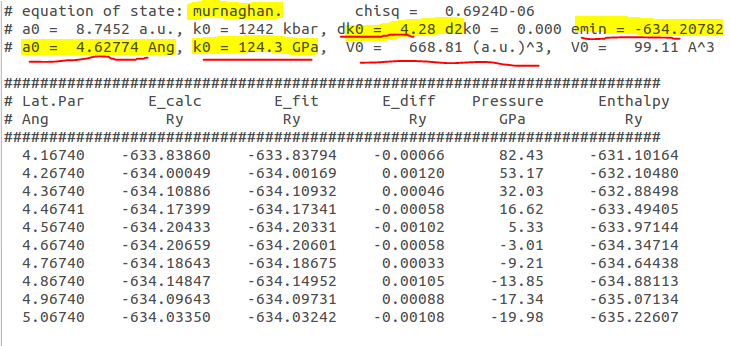# Bulk Modulus calculation using Quantum ESPRESSO

### Tutorial

Quantum ESPRESSO has a package called ev.x that can be used to calculate the bulk modulus of a material. ev.x basically offers calculation of 4 equation of states, namely: These equation of states give the equilibrium volume (lattice constant in case of cubic) as well as the bulk modulus and it’s first derivative.

The procedure is pretty simple. You first need to calculate the energy of the system at different volumes of unit cell. So you would basically need to run several SCF calculations at different values of lattice parameters (thereby different volumes) and note down the corresponding energies in a text file. For cubic systems you can have the lattice parameter in the first column and the Energy in the second column, while for non-cubic systems, you’d note down the volumes in the first column.

A typical file would look like this:

Let’s say this text file is called: volvsE.txt

Now, we can use this to get the Murnaghan  equation of state which is very popular among the scientific community.
Murnaghan EOS is given by:$\boxed{E(V)=E(V_0)+\frac{B_0V}{B'_0}\left[\frac{\left(V_0/V\right)^{B_0'}}{B'_0-1}+1\right]-\frac{B_0V_0}{B_0'-1} }$

Now you just need to run ev.x from the command-line (terminal) and supply inputs to the following prompts:
 [email protected]:~$/home/manas/qe-6.1/bin/ev.x Lattice parameter or Volume are in (au, Ang) > Angstrom Assuming Angstrom Enter type of bravais lattice (fcc, bcc, sc, noncubic) > sc Enter type of equation of state : 1=birch1, 2=birch2, 3=keane, 4=murnaghan > 4 Input file > volvsE.txt Output file > eos  Once, the calculation is done you should have file (eos.txt) that looks like this:Example output file generated from ev.x This contains information of the equilibrium volume, bulk modulus and it’s derivative and the minimum energy. You can use the above generated data to create a neat plot of the equation of state (as you’d see in many publications), using gnuplot. The following shell script, plots the calculated data-points from file volvsE.txt as well as the fitted equation of state curve. [NOTE: This shell script is for cubic systems only. You’d need to modify the code to implement it for non-cubic systems] Create a file called script.sh and add the following code to it: #!/bin/bash a="$1"
k="$2" dk="$3"
emin="$4" V=$(echo "$a*$a*$a"|bc) echo " set terminal png size 1000,500 set output 'EOSplot.png' set xlabel 'Volume (Ang^3)' set ylabel 'Energy (Ry)' set title 'Equation of state' #set key box linestyle 1 f(x)= "$emin"+(x*10**(-30)*"$k"*10**9/"$dk"*(("$V"/x)**"$dk"/("$dk"-1)+1)-"$k"*10**9*"$V"*10**(-30)/("$dk"-1))/(1.6*10**(-19)*13.6)
plot f(x) w l title 'Murnaghan EOS fit', 'volvsE.txt' u 1:2 w points pointtype 7 pointsize 2 title 'Calculated data-points'
set terminal postscript enhanced color solid 22
set output 'EOSplot.eps'
set xlabel 'Volume (Ang^3)'
set ylabel 'Energy (Ry)'
set title 'Equaiton of state'
set autoscale
plot f(x) w l title 'Murnaghan EOS fit', 'volvsE.txt' u 1:2 w points pointtype 7 pointsize 2 title 'Calculated data-points'
set term x11" >EOSplotScript.p



Now save the file and open the terminal and run the command:
chmod u+x script.sh

Now simply run the script by providing the four arguments, in the following order: lattice parameter, bulk modulus, derivative of BM, and minimum energy.

Example: ./script.sh 5.5 81 3.9 -588.656

The above command will create a gnuplot script file called: EOSplotScript.p

This can then be run using gnuplot using the following command:
gnuplot ./EOSplotScript.p

When you run this command, it will generate a PNG image as well as an EPS file.

The output looks like this:

### References

 F.D. Murnaghan, Proceedings of the National Academy of Sciences 30, 244 (1944).## 7 thoughts on “Bulk Modulus calculation using Quantum ESPRESSO”

1. can you help to calculate the bulk modulus of an alloy?

1. Hi there
https://youtu.be/G375GDFqpN4

And let me know if you still have a problem

2. I am working on DFT. Can you help me to know the adsorption study using quantum espresso and let me know how to fix the layers of the slab.

3. Hello Dear Manas
Thanks for this instructive video.
The main thing in your calculation which was not mentioned by you is that for using EOS we have to change the volume in the crystal and put the “relax” mode calculation, not scf calculation.
Because the energy obtained in a specific volume is not true. So, the we need to put “relax” mode for each volume.

Best Regards
Vahid

4. Hello Dear Manas
Thanks for this instructive video.
The main thing in your calculation which was not mentioned by you is that for using EOS we have to change the volume in the crystal and put the “relax” mode calculation, not scf.
Because the energy obtanied in a specific volume is not true, since the atoms have not been relaxed.
So, for each volume we need to put “relax” mode in our calculations.

Best regards
Vahid

1. Sorry, but I don;t think I agree with that.
Actually, this method is hardly used to calculate the crystal parameters nowadays. The only use is to calculate the bulk modulus. The best way to obtain the crstal parameters and atomic coordinates owuld be to run a vc-relax calculation as you must already know.
But then running a relax calculation for every volume won;t give very meaningful results. You see, when the volume is changed the atomic positions (in crystal/fractional coordinates) are still expected to be the same, for the above formula to work correctly. As only then can the energies for different volumes be compared. You see this way the only two things that are changing are volume and energies. But if I start using relax for every volume, then it could be that the atoms relax to be in very strange positions for very large or very smals volumes, which may affect the results. Usually, if you expect an atom to be at 0.33 fractional coordinate in x-axis, then just use that for all the volumes.

5. hi my friend manas..
i have done CPMD in quantum espresso calcium ion with a number of water molecules

can u suggest some way to visualize the trajectories and simulation analyze the radial distribution function?